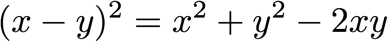Manifold BlogA common problem that comes up in machine learning is needing to find the l2-distance between two sets of vectors. For example, in implementing the k-nearest-neighbors algorithm, we have to find the l2-distance between the a set of test vectors, held in a matrix X (MxD), and a set of training vectors, held in a matrix X_train (NxD). Our goal is to create a distance matrix D (MxN) that contains the l2-distance from every test vector to every training vector. How can we do this efficiently?

Single Loop

There is the really stupid way of constructing the distance matrix using using two loops—but let’s not even go there. That is known inefficient. The first thing I tried was to use a single loop over the test vectors. The code is below. The logic is simple: for each test vector, I subtract it from the entire training matrix. I use a trick called NumPy addition broadcasting. As long as the dimensions match up, NumPy knows to do a row-wise subtraction if the element on the right is one-dimensional. After this subtraction, I simply element-wise square and sum along the column dimension to get a single row of the distance matrix for test vector i.

With M=500 (test vectors) and N=5000 (training vectors) running, this function takes about 50.8 seconds. Too long!

``def compute_distances_one_loop(self, X):``    dists = np.zeros((num_test, num_train))``    for i in xrange(num_test):``        dists[i, :] = np.sum((self.X_train - X[i, :])**2, axis=1)``    return dists`    `1 loops, best of 3: 50.8 s per loop``

No Loops

I need to vectorize more. How? After some Googling and old-fashioned pen and paper, I figured out a better way. The “trick” is to expand the l2 distance formula. For each vector x and y, the l2 distance between them can be expressed as:To vectorize efficiently, we need to express this operation for ALL the vectors at once in NumPy. The first two terms are easy — just take the l2 norm of every row in the matrices X and X_train. The last term can be expressed as a matrix multiply between X and transpose(X_train). NumPy can do all of these things super efficiently.

The final code is below. It took a second to get it right. The trickiest part was figuring out how to transpose the last row vector that comes out of `np.sum()` back into a column vector so the sum broadcast operation works easily, i.e., using `[:, np.newaxis]` (thank you, StackOverflow). Without that trick, I was transposing the larger matrix and transposing back at the end.

``def compute_distances_no_loops(self, X):``    dists = -2 * np.dot(X, self.X_train.T) + np.sum(self.X_train**2,    axis=1) + np.sum(X**2, axis=1)[:, np.newaxis]``    return dists``
``1 loops, best of 3: 190 ms per loop``

So, what does it buy me? It takes only 190 ms for this code to run on the same example above. That’s a speedup of over 267 times! A dirty vectorization trick—but well worth the effort. Now I don’t have to sit around while my distance matrix is computed.

Editor's Note: This blog post was originally published on Medium. It has been lightly edited for this space.

Topics: Data science